Counting the number of ways to express a number as sum of whole numbers has long interested mathematicians. Such sums are called partitions of n and they have many practical applications.

# Integer Sums

The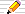icon means there is a Things to do section of questions to start your own investigations and # means the number of. The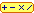calculator icon indicates that there is a live interactive calculator in that section.

## Sums in their different forms

How many ways can you write a number as a sum?
That is basically what we are looking at on this page, the various forms and what happens if we put extra conditions on the sum, such as it must only be odd numbers that are used.
These are called partitions of n but other words are used too depending on what we mean by the same sum. For instance is 2+1 the same sum as 1+2 or do we want to count it as a different answer. If a different order of the same numbers gives a different answer, we will call them compositions of n (following D E Knuth and G E Andrews and others) and if the order of the numbers in the sum does not matter so that different orders of summands are counted as the same sum, then we call them partitions of n.

Here we are interested in partitioning a number meaning to write a positive whole number as a sum of smaller positive whole numbers, e.g:
9 = 5 + 2 + 1 + 1

### Rods

There are also different ways that we can classify our partitions. A useful illustration is the rods of different lengths that children play with.
We assume there are as many of each length as we need, each length having its own unique colour, from single red ones (length 1 which are cubes), length 2 are orange, length 3 are blue and so on as shown in this table:
 length 1length 2length 3length 4length 5length 6... ...
With this scenario, we are interested in putting rods in a line to make a given length. We are sometimes interested in the order of the colours, and other times just the number of each kind of rod used. Sometimes we will want any colour to be used no more than once in each line.
Such diagrams will turn out to be very useful in proving results connecting different kinds of partitions later.

### Partitions and Compositions

Both of these names have many uses in mathematics.
The word partition can mean partitioning a set into subsets (as in partitioning a computer hard-drive so that various parts are used for various separate and distinct purposes). Also a composition in maths can apply to combining two functions into one by applying two or more functions in order one after the other.
Neither of these uses are the subject of this webpage!
So let's be a little more precise about how these two words as used in Number Theory, though be careful because other mathematicians use different words.
Some just use the single word partition but then qualify it by saying "of distinct numbers" and "where the order is important".
Partitions of an integer N (the order in the collection does not matter)
• A set of whole numbers (a collection of unique numbers, no repetitions) whose sum is N, where the order does not matter.
In terms of the rods, we are interested in the number of each colour used.
For instance, the partitions of 3 are [1,1,1], [1,2] and  only. [2,1] is the same partition (contains exactly the same elements) as [1,2].
• If numbers may be repeated in the sum then the collection is called a multi-set or bag.
Here we allow [1,1,1] but [1,2] is the same collection as [2,1].
Compositions of an integer (the order of the parts in the sum is important).
A sequence or list of whole numbers whose sum in N, where the order does matter and numbers may be repeated in the sequence.
These are sometimes called ordered partitions (as in MathWorld) meaning that the only collections used are those with the parts "in order" but we will use the term compositions.
Other names for a sequence are:
a list and
as a generalisation of double, triple, quadrulple, ... they are also called tuples.
Again we ask if items are allowed to be repeated in a composition sequence. If not, the parts of any composition must be unique, distinct.
• Compositions without repetitions (distinct or unique parts)
For instance, the compositions of 3 are [1,2], [2,1] and  because the order is now important. If repetition is not allowed then we must exclude [1,1,1].
For the rods, the sequence of colours along the line is important so that [1,2] is red-orange whereas [2,1] is orange-red so they are different arrangements.
• Compositions with repetition allowed.
In these collections the order matters (a different ordering of the components gives a separate composition) but items can be repeated.
Here all 4 sequences that sum to 3, namely [1,1,1], [1,2],[2,1] and  are all counted and are all different.
Omar Pol has a wonderful image of partitions (bags) using rods: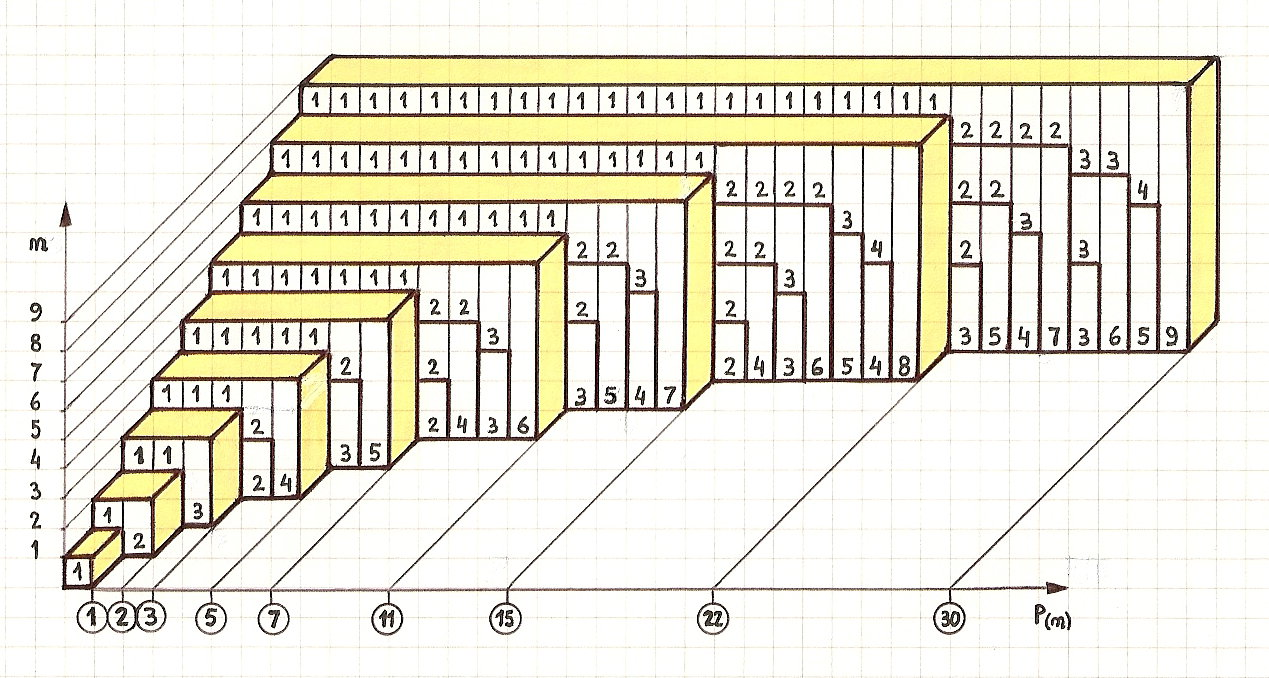### Notation

#### Brackets {} and []

Sets
are traditionally written using curly brackets: {3,1,2}.
Items are listed once since in a set the property of interest is if a number is in the set or not so repetitions are irrelevant and no item is listed more than once.
For the rods illustration, no colour of rod may be used more than once and it is the collections of colours that we want to count.
Lists (sequences, series)
are written in a variety of bracket styles, sometimes inside round brackets () or square [] or angled brackets <>.
We will use [] on this page for a list where the order matters in the list.
There are 3 lists of distinct numbers that sum to 3, namely , [1,2] and [2,1].
We will also include [1,1,1] if we are allowed to repeat items in the list.
For a multiset (bag)
we are now not only interested in whether or not an element is in the collection but also the frequency of an item is needed.
There are a variety of notations for a multi-set but here we will still use curly brackets.
There are 3 multisets of integers that sum to 3: {3}, {1,2} and {1,1,1}.

To summarise and for comparison, we have:

Repetition?allowednot allowed
Order mattersComposition
list
Composition
list
Order does not matterPartition
bag
Partition
set

SumRepetition allowedNo Repetitions
Partitions
Bags
CompositionCompositionPartition
set
2{1,1},{2}[1,1],{2}
3{1,1,1},{1,2},{3}[1,1,1],[1,2],[2,1],[1,2],[2,1],{1,2},{3}
4{1,1,1,1},{1,1,2},{1,3},{2,2},{4} [1,1,1,1},[1,1,2],[1,2,1},[1,3],
[2,1,1],[2,2],[3,1],
[1,3],[3,1],{1,3},{4}

#### The p(n|...) notation

Partitions can involve repetitions or not. Compositions are sometimes called ordered partitions too. A common notation is to use p( n | conditions ) where we note the conditions on a partition after the vertical line.
The parts of the partition are therefore pi with p1 being the first number in the partition and often we ues pk for the last so that there are k numbers in the partition; it has length k.
Partitions mean we list the parts in order (smallest to largest or largest to smallest):
Partitions with repetition: p(n | p1 ≥ p2 ≥ ... ≥plast)
Partitions without repetition: p(n | p1 > p2 > ... >plast)
Compositions the order counts so we do not include the inquality conditions above.

#### The product notation

Sometimes, writing a partition as {1,1,1,1,1,1,3,3,3,3,3} would be easier if we showed the frequency of the parts.
A shorthand notation that is used is based on how multiplication and powers are written even though we are looking at sums. The superscript means the frequency of the number.
So the example partition here has six 1s and five 3s and would be written 16 35
The short-notation partition ap bq cr... has sum a p + b q + c r + ...

### A source of confusion: sorted or ordered

One source of confusion is that with the collections where the order does not matter, in fact it does!
By "order does not matter" we mean that reordering the elements will not produce a different answer; any order is equivalent to any other order provided the frequencies of the elements are the same.
Among the Partitions of 7 we have:
[1,1,2,3] which is the same as [1,2,1,3] and [1,1,3,2] and [3,2,1,1] and some others Show the others?
[1,2,3,1],[1,1,3,2],[1,3,1,2],
[2,1,1,3],[2,1,3,1],[2,3,1,1],
[3,1,2,1],[3,1,1,2]
12 in all since we choose any of the 4 for the first in the list, leaving any of the remaining 3 for second place, any of the final 2 for third place (and so the final element is in fourth place) giving 4×3×2=24 ways, except that there are two 1s so we have counted each collection twice ( the second having the two 1s "invisibly" swopped).
Thus there are
 4×3×2 = 12 2
different permutations of 1 + 1 + 2 + 3.
since each of these contains two 1s, one 2 and one 3 and these are the same partition of 7.
So one simple way to remove the duplicates is to filter the possible permutations of the items and only include those that are sorted, already in order, that is only count those in increasing order say. Except that, where we may have values repeated, we allow equal items. This is usually written as "non-decreasing" instead of "increasing" to allow for the equal items.

In the example permutations above, only the first is chosen as the representative of the rest because it has its elements in order.

So "order does not matter" is equivalent to "counting only those lists that are already sorted or ordered"!
"Order does matter" means that every collection counts, whether it is sorted into some order or not!

This is important particularly when writing a program to find various partitions and compositions but often leads to confusion on first meeting partitions and compositions.

### Mathematical Definitions

We can use the idea of a sum to define our various kinds of partitions:
Each sum is a list a1, a2, a3, ...ak of k terms or parts, where all the parts ai are positive whole numbers.
The main condition is that a1 + a2 + ... + ak = n.
Usually we want all the variables (parts) to be positive (non-zero) whole numbers.
Notation:
The set of natural numbers: {0,1,2,3,...} is
If we want to exclude 0 and just indicate the strictly positive integers {1,2,3,...}, we use +
The symbol , an upside down "A", means for all so
i=1..k some condition
means the condition applies to all the indices i from i to k.
Partition bag or Partition multi-set
Any solution of a1 + a2 + ... + ak = n i=1..k, ai+ and 1 ≤ a1 ≤ a2 ≤ ... ≤ ak ≤ n
The possibility of equality between each pair of terms allows for terms to be repeated.
Partition set (distinct parts)
Any solution of a1 + a2 + ... + ak = n i=1..k, ai+ and 0 < a1 < a2 < ... < ak < n
Here all the ai are unique and there is no equality allowed between items:
no value appears more than once in the partition. This is also the condition that the partition forms a set (no repetitions):
ai = aj if and only if i = j (for 1≤i<j≤k)
Composition of n with repetitions
Any solution of a1 + a2 + ... + ak = n, i=1..k, ai+
Since each term in the sum is separately named, the order in the sum matters and terms may be repeated.
Composition sequence without repetitions
Any solution of a1 + a2 + ... + ak = n i=1..k, ai+ and ai = aj if and only if i = j
The second condition ensures no numbers are repeated in a partition but the a's can be in any order. We are considering the numbers in sequence here, not as a set.

## How many?

There is one solution to a collection of positive numbers whose sum is 0, namely the empty sequence [] or the empty set {}.

### Compositions (Sequences with repetitions)

These are sequences that sum to a given value and items in the sequence may be repeated. Here we use the short notation where the number of repetitions of a number is indicated as a superscript, a "power" and all the numbers are single digits in the following tables.
nSequences Count
1 11
2 2, 122
3 3, 12, 21, 134
4 4, 13, 22, 122, 31, 121, 212, 148
5 5, 14, 23, 123, 32, 122, 212, 132, 41, 131, 221, 1221, 312, 1212, 213, 1416
The number of compositions (sequences with repetition allowed) of a number n is the easiest to find a formula for.
Do you recognize the counts in the final column?
But why? Can you find a reason?
We can write the compositions as follows:
... write n 1s in a row then put a "," or a "+" between them in all possible ways. The composition is found by performing the additions:
 [1, 1, 1, 1] 1 , 1 , 1 , 1 3 commas, 0 additions [1, 1, 2] 1 , 1 , 1 + 1 2 commas, 1 addition [1, 2, 1] 1 , 1 + 1 , 1 [2, 1, 1] 1 + 1 , 1 , 1 [1, 3] 1 , 1 + 1 + 1 1 comma, 2 additions [2, 2] 1 + 1 , 1 + 1 [3, 1] 1 + 1 + 1 , 1  1 + 1 + 1 + 1 no commas
There are two choices for each of the n-1 spaces between the n 1s so there are 2n-1 compositions of n allowing repetitions.
The number of compositions of n allowing repetitions in a composition: 1, 1, 2, 4, 8, 16, 32, 64, 128, 256,... A011782.
The Calculator below will provide approximations to the number of compositions (sequences with repetition) for very large n up to around n=1000 before even the approximations become too large for JavaScript when they exceed 1.7×10308).

### Compositions (sequences without repetitions)

Sequences with no repeated elements and with a given sum n.
They are also called compositions with distinct parts .
nSequences without repeated itemsCount
1 11
2 21
3 3, 12, 213
4 4, 13, 313
5 5, 14, 41, 23, 325
6 6, 15, 51, 24, 41, 123, 132, 213, 231, 312, 32111
The counts are 1,1,3,3,5,11,13,19,27,57, ... A032020
Counting compositions without repetition where ~ indicates an approximate number:
nCount
1057
50107 59321
100109 38301 18491
500~9.184809494×1037
1000~1.541212300×1060
2000~3.042018006×1094
10000~4.355395751×10259

### Partitions (sets with repetition: multi-sets or bags)

nBags (sets with repeated items)Count
1 {1}1
2 {2} {1,1}2
3 {3} {2,1} {1,1,1}3
4 {4} {3,1} {2,2} {2,1,1} {1,1,1,1}5
5 {5} {4,1} {3,2} {3,1,1} {2,2,1} {2,1,1,1} {1,1,1,1,1}7
6 {6} {5,1} {4,2} {4,1,1} {3,3} {3,2,1} {3,1,1,1} {2,2,2} {2,2,1,1} {2,1,1,1,1} {1,1,1,1,1,1}11
7 {7} {6,1} {5,2} {5,1,1} {4,3} {4,2,1} {4,1,1,1} {3,3,1} {3,2,2} {3,2,1,1} {3,1,1,1,1} {2,2,2,1} {2,2,1,1,1} {2,1,1,1,1,1} {1,1,1,1,1,1,1}15
8 {8} {7,1} {6,2} {6,1,1} {5,3} {5,2,1} {5,1,1,1} {4,4} {4,3,1} {4,2,2} {4,2,1,1} {4,1,1,1,1} {3,3,2} {3,3,1,1} {3,2,2,1} {3,2,1,1,1} {3,1,1,1,1,1} {2,2,2,2} {2,2,2,1,1} {2,2,1,1,1,1} {2,1,1,1,1,1,1} {1,1,1,1,1,1,1,1}22
9 {9} {8,1} {7,2} {7,1,1} {6,3} {6,2,1} {6,1,1,1} {5,4} {5,3,1} {5,2,2} {5,2,1,1} {5,1,1,1,1} {4,4,1} {4,3,2} {4,3,1,1} {4,2,2,1} {4,2,1,1,1} {4,1,1,1,1,1} {3,3,3} {3,3,2,1} {3,3,1,1,1} {3,2,2,2} {3,2,2,1,1} {3,2,1,1,1,1} {3,1,1,1,1,1,1} {2,2,2,2,1} {2,2,2,1,1,1} {2,2,1,1,1,1,1} {2,1,1,1,1,1,1,1} {1,1,1,1,1,1,1,1,1}30
1,2,3,5,7,11,15,22,30,42, ... A000041
The number of partitions (multi-sets with repetitions) of n is usually denoted by p(n).
np(n)
1042
100 1905 69292
200 397 29990 29388
500 23 00165 03257 43239 95027
The number of digits in a value x is calculated by 1+log10(x) Here are some graphs of the number of partitions (bags) to show that the number increases very rapidly:
1..20:
1..30:
1..100:
1..500:
Number of digits, 1..500: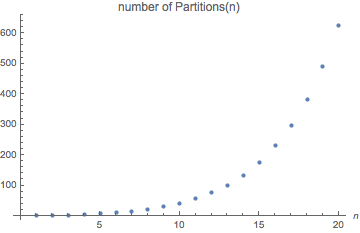The Calculator below will provide approximations to p(n) for very large n up to around n=76000 before even the approximations become too large JavaScript (they exceed 1.7×10308)!

### Partition sets

nSetsCount
1 {1}1
2 {2}1
3 {3} {2,1}2
4 {4} {3,1}2
5 {5} {4,1} {3,2}3
6 {6} {5,1} {4,2} {3,2,1}4
7 {7} {6,1} {5,2} {4,3} {4,2,1}5
8 {8} {7,1} {6,2} {5,3} {5,2,1} {4,3,1}6
9 {9} {8,1} {7,2} {6,3} {6,2,1} {5,4} {5,3,1} {4,3,2}8
10 {10} {9,1} {8,2} {7,3} {7,2,1} {6,4} {6,3,1} {5,4,1} {5,3,2} {4,3,2,1}10
The counts are 1,1,2,2,3,4,5,6,8,10, ... A000009
There are 444793 Partition-sets for 100 and 86 35565 79574 41551 61506 for 1000.

### Partitions and Compositions into parts

If we specify that our partitions and compositions are to be of a given length k, we are saying that they must contain exactly k non-zero numbers or have k parts.

#### Compositions into parts

We can further classify compositions by how many numbers are in the list:
There are 23 = 8 compositions of 4:
Compositions of 4 with 4 parts: [1,1,1,1]
Compositions of 4 with 3 parts: [2,1,1], [1,2,1], [1,1,2]
Compositions of 4 into 2 parts: [1,3], [3,1], [2,2]
Compositions of 4 into 1 part: 

### Count comparison for partitions and compositions

Here is a table of the number of partitions and compositions for small values and some large ones
nPartitions (sets/bags)Compositions (sequences)
no repswith repsno repswith reps
1 1 1 1 1
2 1 2 1 2
3 2 3 3 4
4 2 5 3 8
5 3 7 5 16
6 4 11 11 32
7 5 15 13 64
8 6 22 19 128
9 8 30 27 256
10 10 42 57 512
20 64 627 1555 524288
30 296 5604 41867 5.369×108
50 3658 204226 10759321 ~5.630×1014
100 ~4.447×105 ~1.905×108 ~1.093×1012 ~6.338×1029
200 ~4.870×108 ~3.973×1012 ~1.011×1020 ~8.034×1059

## Partitions and Compositions Calculator

A Partition:
a set (without repetition of items) or bag (with repetition allowed);
The order of items in the collection does not matter.
Collections are equal only if they contain the same number of each kind of item. Shown using {} brackets;
A Composition:
a sequence (list); shown using [] brackets.
The order in the collection does matter.
Collections are equal only if they contain the exactly same items in the same order
This Calculator uses formulae and recursion to compute exact and for approximate counts when the choices are unrestricted (are all the whole numbers).
NaN means Not a Number and indicates that an approximation formula is not yet implemented here for Compositions without Repetition.
Use the Restricted Partitions calculator below if the choices are restricted or you want only a specific number or range of part lengths.
Integer Sums by length C A L C U L A T O R
 Partitions (sets/bags) Compositions (sequences) with repetitions without repetitions of up to up to solutions partition format: 'powers' ≥ 'powers' ≤ list ≥ list ≤
R E S U L T S       ~ indicates an approximation: Integer sums Restricted partitions Max Prod partitions Divisors' sum

## The remarkable Partition Identities!

Ever since Euler started investigating partitions some remarkable and unexpected connections have emerged. For instance...

### Odds and Uniques

Suppose we list the partitions on n that use only odd numbers:
Partitions with only odd elements: p(n|odd numbers)
n:123 456
Partitions  = 1 [1, 1] = 12 [1, 1, 1] = 13
 = 3
[1, 1, 1, 1] = 14
[1, 3] = 1 3
[1, 1, 1, 1, 1] = 15
[1, 1, 3] = 12 3
 = 5
[1, 1, 1, 1, 1, 1] = 16
[1, 1, 1, 3] = 13 3
[1, 5] = 1 5
[3, 3] = 32
Counts1 1 2 2 3 4
The counts from n=1 onwards are: 1, 1, 2, 2, 3, 4, 5, 6, 8, 10, 12, 15, ...
Another special case is to look at the partitions that are sets, that is, the ones that contain no repeated item: p(n| no repeated parts)
Partitions with only unique elements
n:123 456
Partitions {1} {2} {1, 2}
{3}
{1, 3}
{4}
{1, 4}
{2, 3}
{5}
{1, 2, 3}
{1, 5}
{2, 4}
{6}
Counts1 1 2 2 3 4
The counts from n=1 onwards are: 1, 1, 2, 2, 3, 4, 5, 6, 8, 10, 12, 15, ...
Is this just coincidence?
No, since the match continues for ever. The series is A000009.
The famous Swiss mathematician Euler noticed this and wrote about it in 1748 and we look at this again below.

### The number of 1s and the number of distinct parts

1,1,1,1: 4 1s
1,1,2: 2 1s
1,3: 1 1
2,2: 0 1s
4: 0 1s
Total: 4+2+1+0+0 =
7 1s used
1,1,1,1: number of distinct parts: 1
1,1,2: number of distinct parts: 2
1,3: number of distinct parts: 2
2,2: number of distinct parts: 1
4: number of distinct parts: 1
Total: 1+2+2+1+1 =
7 distinct parts
Some partition multisets contain a 1 (possibly more) as here for 4 where we count the number of 1s used in all the partitions on the left.

If we look at the number of distinct integers used in each of these partitions we have this table on the right.

These counts will always be the same for every n!

### Partition sets and Partition bags of odds

The number of partition on n into distinct parts (sets) is the same as
the number of partitions of n using only odd numbers but with repeats allowed:
n45678910111213141516
#23456810121518222732
Show why
Let's look at example:
7 as a sum of distinct integers in 5 ways: {1,2,4}, {1,6}, {2,5}, {3,4}, {7}.
For each partition we can convert it into a bag (multiset) of odd numbers as follows:
express each part as (sum of powers of 2)×odd where odd is an odd number and its frequency will be the number in binary that precedes it.
{3,4} becomes { (20)×3 + (22)×1}
The numbers in brackets are the frequencies of the odd numbers after the × symbol:
{3,1,1,1,1}.

Check the correspondence with the other (set) partitions of 7:
partition
set
as binary×odd partition (bag)
of odds
1,2,4 (20)×1,(21)×1, (22)× 1 1 + 1+1 + 1+1+1+1
1,6 (20)×1,(21)×3 1 + 3+3
2,5 (21)×1, (20)×5 1+1 + 5
3,4 (21)×3, (22)×1 3 + 1+1+1+1
7 (20)×7 7

Similarly, a bag of odd numbers may be transformed into a set of distinct numbers with the same sum:
1,3,5 is a partition of 9 into odd numbers but none are repeated so it corresponds to the same as a (set) partition.

1+1+1+1+5 is a partition of 9 into odd numbers. This is also (22)×1,(20)×5 so corresponds to 4+5 as a sum of distinct parts.

• Mathematical Gems III Ross Honsberger, Math. Assoc. of Amer. in ch 6 Two Applications of Generating Functions section 1 A Theorem of Euler pages 64-68 which also has a proof of this correspondence

## Diagrams of Partitions: Ferrers Diagrams and proofs-without-words

We can arrange our collection of coloured rods into a line of length n to illustrate each partition on n.
Since we are dealing with sets and bags, we can order the numbers in each partition from largest to smallest.
For example, one partition of 12 is 12 = 6 + 3 + 2 + 1:We could also line the up in a column:or, to better show their structure (lengths), we could use a square grid. Using the same example we have:
or, without colour:
These empty square grid diagrams of a partition are called Ferrers Diagrams or Ferrers boards or Young diagrams. They immediately illustrate a fact about partitions:

### # partitions with largest part k = # partitions with k items

The # symbol (hash) means the number of.
The number of partitions (sets and bags) with k parts is equal to
the number of partitions (sets and bags) with largest part k
By looking at the Ferrers diagram in two ways, can you see why? Show why
The length of the rows are the parts of the partition, e.g.6,3,2,1.
The largest row is the largest number in the partition, in our example it is 6.
By looking at the columns we have a partition with exactly k parts.
In our example there are 6 columns.
Since every diagram can be viewed in these two ways, as rows and as columns, the two counts must be identical: The number of partitions with largest part equal to k (as rows, with k in the longest row) and
The number of partitions with k parts (as k columns).

### # partitions into even parts = # partitions where each part occurs with even frequency

Using the same reasoning we can see that
The number of partitions of n into parts all of which are even numbers is the same as
the number of partitions of n where each part in it occurs an even number of times
Instead of counting the number of squares in the rows of a Ferrers diagram, we can count the number of squares in the columns.
Consider this example of 12 = 6 + 2 + 2 + 2 and it corresponds to 12 = 4 + 4 + 1 + 1 + 1 + 1 where 4 occurs twice and 1 occurs four times, and the frequencies one and four are even numbers.
If all the rows ar of even length then the each column-count will appear an even number of times.

### # self-conjugate partitions of n = # partitions of n with distinct odd parts

The trick of comparing column-count to row-counts has many applications. Here is another:
The conjugate partition of a partition is found by flipping its Ferrers diagram so that the rows become columns and the columns become rows. This is the same as reflecting the shape in the diagonal from top-left to bottom-right. For example:
{6, 3, 2, 1} row-lengths {4,3,2,1,1,1} as column-lengths
corresponds to

If a Ferrers diagram is the same when flipped (that is, counted by columns instead of rows) then the partition is called self-conjugate.

For example: 23 = 6 + 6 + 4 + 3 + 2 + 2 : {6, 6, 4, 3, 2, 2}

Since these self-conjugate diagrams have the same number of rows as columns, we can remove the top row and the leftmost column and we will still have another self-conjugate partition:

The total number of dots on the top row plus the left edge must always be an odd number (we only count the top left corner dot once).
It is now but a short step to see that we can always match a self-conjugate partition with one made with only odd parts by repeatedly stripping off all the top-row-and-left-edges and noting how many dots are removed each time. For example, in the diagram above, we remove 11 (in yellow) and then 9 (in red) and finally 3 (in green) so self-conjugate partition {6, 6, 4, 3, 2, 2} corresponds to the partition of only odd numbers: {11, 9, 3}.

The number of self-conjugate partitions of n is the same as
the number of partitions of n with distinct odd parts.

## Generating Functions

The essential idea here is to relate a series with a polynomial with the the series' numbers as it coefficients, so that si, the ith member of the series is also the coefficient of xi in the polynomial.
Since the series of infinite, so is the polynomial. Such infinite polynomials in a power of a variable (often x) are called power series.
the series: s0, s1, s2, ... ↔ s0 + s1x + s2x2 + ... :the power series
We know a lot about polynomials and when we can find a simple expression for the polynomial then we can deduce more things about the series related to its coefficients.

### The sequence 1,1,1,1,...

This is the basic series and its generating function, let's call it G(x) for now, is
G(x) = 1 + x + x2 + x3 + ...
This is the same as a simple expression involving x:
We express x G(x) is two ways:
x G(x) = x + x2 + x3 + ...
but if we add 1 to the right-hand side we have G(x) again:
1 + x G(x) = G(x)
Now we can rearrange this to find an expression for G(x):
 1 = G(x) − x G(x) 1 = ( 1 − x) G(x) 1 = G(x) 1 − x
so we see that
 1 + x + x2 + x3 + ... = 1 1 − x

#### The decimal fraction for 1/9

Once we put a value for x into a generating function, we need to be careful about whether the series converges or not. We will not pursue this further on this page but it is an important condition.
If we let x=1/10=0.1 in the power-series for 1/(1-x) we have
1 = 1 = 10 = 10
1 − x
 1 − 1 10
10 − 1 9
and we also have
1 + x + x2 + x3 + ... = 1 + 0.1 + 0.01 + ... = 1.11111...
and 1+1/9 is indeed 1.11111... .

Since x can be any number, not just 1/10, then with x=1/2 we have the binary value 1.11111...2 = 2
With x=1/3, 1.11111...3 = 3/2 = 1 + 1/2. This is a quick proof that

 1 + 1 + 1 + 1 + ... = 1 3 32 33 34 2

#### The decimal fraction for 1/98

Another example uses x=1/100 in the power-of-two generating function which we find by replacing x by 2x in the GF above:
 1 + 2 x + (2 x)2 + (2 x)3 + (2 x)4 + ... = 1 1 - 2 x
First we multiply by x, to get rid of the initial "1" in order to get a purely decimal fraction (less than 1) and then let x = 1/100:
x + 2 x2 + 22 x3 + 23 x4 + ...
= .01 + .00 02 + 0.00 00 04 + 0.00 00 00 08 + 0.00 00 00 00 16 + ...
= 0.01 02 04 08 16 ...
Looking at the fraction form with x=1/100 we have:
 x = 1/100 = 1 = 1 1 - 2 x 1 - 2/100 100 - 2 98
and this explains why the decimal fraction for 1/98 is the powers-of-two in blocks of 2 digits!

### Number of partitions (sets)

Another easy association between a series and a generating function (power series) is the series that counts the number of partition sets with sum n (partitions without repetition, partition sets):
 n # 1 2 3 4 5 6 7 8 9 10 11 12 ... 1 1 2 2 3 4 5 6 8 10 12 15 ... A000009
Here we will see that it the counts are just the coefficients in the (infinite) expansion of
(1 + x)(1 + x2)(1 + x3)(1 + x4)(1 + x5)...
which we will see is the same as
1 + x + 2 x2 + 2 x3 + 3 x4 + 4 x5...
To see this, we can find only one way to get the coefficient 1, by picking the "1" from each of the brackets.
There is similarly only one way to get x2, by choosing the 1 frmo all the brackets and the x2 from the (1 + x2) bracket.
When it comes to x3, we can choose (1 + x)(1 + x2) and 1 from all the other brackests
OR 1's from all except the (1 + x3) brackets, since 3 is 1+2 and 3.
For 4, since 4 = 1+3 where are two ways of choosing the terms. This is because each powers of x can only be used once so there are no repetitions, and also there is essentially only one order to choose each collection of x's that multiply to x4. The Generating Function for the number of partition (sets) for n is
 ∞ (1 + x)(1 + x2)(1 + x3)(1 + x4)(1 + x5)... = Π (1 + x)i i = 1
where the capital Pi symbol Π stands for Product, meaning we multiply all the terms (1 + x)i for values of i from 1 up to infinity.

#### The General Pentagonal Numbers

Here we make a list of the number of partitions of n (sets without repetition) for n from 1 to 20 and count the number of partitions of n that are of even length and the number of odd length. For some numbers, these are equal, but not all:
Counts of Partitions of n without repetition by number of parts (even or odd length)
n1234567891011121314151617181920212223242526
p(n)11223456810121518222732384654647689104122142165
# even length001122334567911111619232732384552617183
# odd length111112234568911121619232732384452617182
difference+1+1 -1 -1 +1 +1 -1 -1
For which numbers are they not equal?
For n = 1, 2, 5, 7, 12, 15, 22, 26, 35, 40, .... A001318
These include the pentagonal numbers:
 side shape size 1 2 3 4 5 6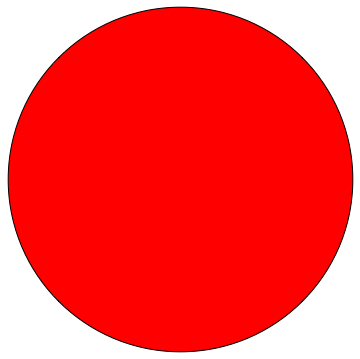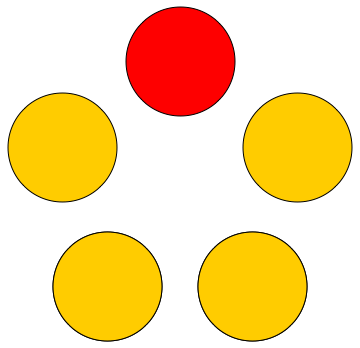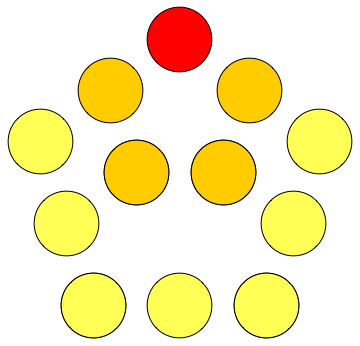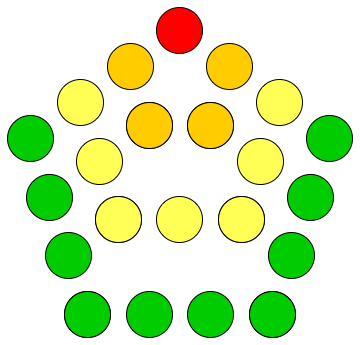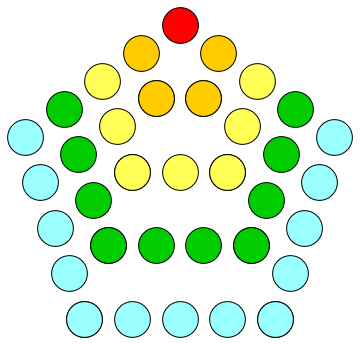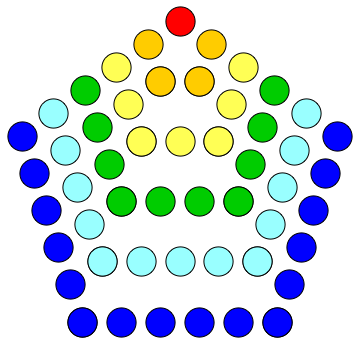1 5 12 22 35 51

1, 5, 12, 22, 35, 51, ... which is A000326
with the formula that the k-th is (3 k2 − k)/2 with k = 1, 2, 3, ....
The extra numbers, 2, 7, 15, 26, ... and just those with the same formula but k is negative. Also, the k-th in this list is k more than the k-th in the Pentagonal number list. They given by the expression n = (3 k2 ± k)/2, for k=1,2,3,...
The combined series is called the General Pentagonal Numbers: 1, 2, 5, 7, 12, 15, 22, 26, ... A001318.

In our table of counts of odd length and even length partitions, the sign of the difference between them depends on k.
If k is odd the difference is +1; if k is even, the difference is -1.
Show the k values in the Table above
This was implied (known) by Euler but not stated by him as such. (See Dickson Vol II, page 113.)

An easy way to produce the general pentagonal numbers in order is to note that (it is easy to prove that) they are one third of the Triangle numbers that are multiples of 3

 n T(n)= ÷3 n(n+1)/2 1 2 3 4 5 6 7 8 9 10 ... 1 3 6 10 15 21 28 36 45 55 ... - 1 2 - 5 7 - 12 15 - ...
Another way of looking at the General Pentagonal Numbers is that those with a positive value of k (the pentagonal numbers) are a square with a triangle on top sharing a common side: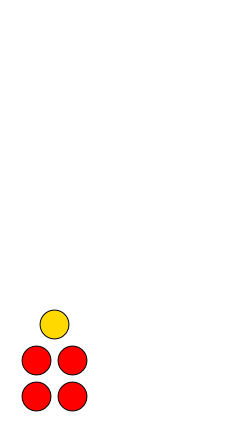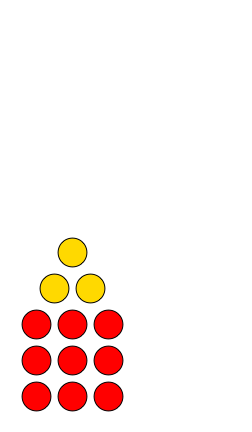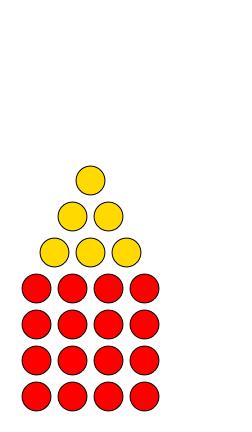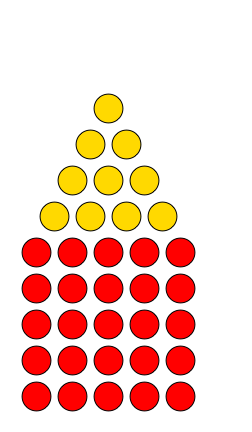Pentagonal Numbers
A000326
1+0=14+1=59+3=1216+6=2225+10=35
 k2 + k ( k − 1) = 3 k2 − k 2 2
12+33+4+54+5+6+75+6+7+8+9k + (k+1) + ... + (2k - 1)
but those with a negative value of k do not share the common side: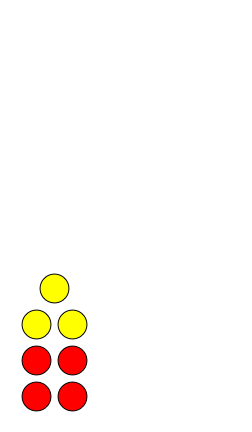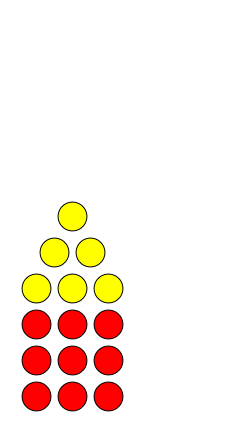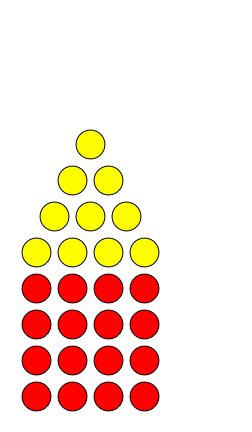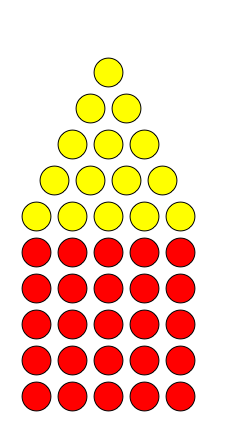Second Pentagonal Numbers
A005449
1+1=24+3=79+6=1516+10=2625+15=40
 k2 + k ( k + 1) = 3 k2 + k 2 2
23+44+5+65+6+7+86+7+8+9+10(k+1) + (k+2) + ... + 2k

### Number of partitions with repetition (bags or multi-sets)

This time we can repeat any number in our collection whose sum is n.
 1 + x + x2 + x3 + ... = 1 1 − x
This essentially relates to choosing 1 (the coefficient) and number of times (as given by the power of x).
If we replace x by x2 we have
 1 + x2 + x4 + x6 + ... = 1 1 − x2
which gives us powers which are multiples of 2. Similarly for multiples of 3
 1 + x3 + x6 + x9 + ... = 1 1 − x3
So we make a partition of n which allows repetitions, we choose one term from the first series to represent the number of 1s or choose 1 itself if we do not want to use any 1's;
choose one term from the second series to represent 2s, and so on, provided that the product of all the terms used is xn! We therefore have
 number of partition bags with sum n are the coefficients of 1 1 1 1 ... (1 - x) (1 - x2) (1 - x3) (1 - x4)

#### A Historical note

The connection with these generating functions is why the term partition is linked with this particular form of partition: a multiset (bag) with repetition allowed.
The connection goes back to Euler and intrigued Ramanujan and many others as it is rich in interesting connections and theorems.
If we let p(n) denote the number of partitions (bags with repetition allowed) then Ramanujan noticed and proved that
• p(5k + 4) is always a multiple of 5 for all k
 k n=5k+4 p(n) p(n)/5 0 1 2 3 4 5 6 7 ... 4 9 14 19 24 29 34 39 ... 5 30 135 490 1575 4565 12310 31185 ... 1 6 27 98 315 913 2462 6237 A071734
• p(7k + 5) is always a multiple of 7 for all k
 k n=7k+5 p(n) p(n)/7 0 1 2 3 4 5 6 7 ... 5 12 19 26 33 40 47 54 ... 7 77 490 2436 10143 37338 124754 386155 ... 1 11 70 348 1449 5334 17822 55165 A071746
• p(11k + 6) is always a multiple of 11 for all k
 k n=11k+6 p(n) p(n)/11 0 1 2 3 4 5 6 7 ... 6 17 28 39 50 61 72 83 ... 11 297 3718 31185 204226 1121505 5392783 23338469 ... 1 27 338 2835 18566 101955 490253 2121679 A076394
S Ramanujan (in Proceedings of the London Mathematical Society, Series 2, vol 16 (1918) pages 352-4) published some results about p(n), the number of partitions (bags with repetitions allowed) of n: (a ≡ 0 (mod b) means a is a multiple of b)
p(5m - 1) ≡ 0 (mod 5)
p(7m - 2) ≡ 0 (mod 7)
p(35m + 19) ≡ 0 (mod 35)
p(25m - 1) ≡ 0 (mod 25)
p(49m - 2) ≡ 0 (mod 49)
which he derived from
 p(4) + p(9)x + p(14)x2 + ... = 5 ( (1 - x5)(1 - x10)(1 - x15) ...)5 ( (1 - x )(1 - x2 )(1 - x3 ) ... )6

 p(5) + p(12)x + p(19)x2 + ... = 7 ( (1 - x7)(1 - x14)(1 - x21) ...)3 ( (1 - x )(1 - x2 )(1 - x3 ) ... )4 + 49x ( (1 - x7)(1 - x14)(1 - x21) ...)7 ( (1 - x )(1 - x2 )(1 - x3 ) ... )8
Ramanujan thought he had found a more general pattern based on a large table of partitions and on his observations, but he was slightly wrong.
It was quite a few years later (1967) before a correct generalisation was found and proved by A O L Atkin:
Theorem: If 24 n leaves a remainder of 1 when divided by 5a 7b 11c then p(n) is a multiple of 5a 7(b+2)/2 11c
Andrews (Theory of Partitions, page 160) gives an example to show why this new form was needed and not, as Ramanujan conjectured, the simpler ... then p(n) is a multiple of 5a 7b 11c:
Let n=243 and a=0,b=3,c=0.
Then 24 n = 24×243 does have a remainder of 1 when divided by 73 but p(n) = p(243) = 133978259344888 which is not a multiple of 73.
The modified (correct) theorem excludes this case because (b+2)/2 is not an integer when b=3.

### Number of compositions (sequences with repetition)

We have already seen that there are 2n-1 composition sequences with sum n if we allow repetitions in a sequence. So how do we find the generating function for 1, 1, 2, 4, 8, 16, ... ?

We can start from the powers-of-2 generating function:

 1 + 2 x + 22 x2 + (2 x)3 + (2 x)4 + ... = 1 1 − 2 x
First we subtract 1
 2 x + 22 x2 + (2 x)3 + (2 x)4 + ... = 1 − 1 = 1 − (1 − 2x) = 2 x 1 − 2 x 1 − 2 x 1 − 2 x
Now we can halve this to get all but the first term that we need:
 x + 2 x2 + 22 x3 + 23 x4 + ... = x 1 − 2 x
 1 + x + 2 x2 + 22 x3 + 23 x4 + ... = x + 1 = x + 1 − 2 x = 1 − x 1 − 2 x 1 − 2 x 1 − 2 x

### Number of compositions (sequences without repetition)

1, 1, 1, 3, 3, 5, 11, 13, 19, 27, 57, ... A032020
This it seems is not possible as a simple power series!

### Application: giving coins in change

Suppose a shopkeeper has a cash drawer with lots of £1, £2 coins and £5 notes.
A customer needs change to the value of £12. In how many ways can the shopkeeper give £12 using only those denominations in the cash drawer?
Since we can repeat coins and the order of coins does not matter in the change, we have paritions with repetition or multi-sets or bags (of change).
 The repetition of 1s has the generating function 1 = 1 + x + x2 + x3 + ... 1 − x The repetition of 2s has the generating function 1 = 1 + x2 + x2×2 + x3×2 + ... 1 − x2 The repetition of 5s has the generating function 1 = 1 + x5 + x2×5 + x3×5 + ... 1 − x5

 The GF for the number of ways of giving change is therefore 1 (1 − x)(1 − x2)(1 − x5)
We are interested in the coefficient of x12 in this GF which begins:
1 + x + 2 x2 + 2 x3 + 3 x4 + 4 x5 + 5 x6 + 6 x7 + 7 x8 + 8 x9 + 10 x10 + 11 x11 + 13 x12 + ...
and we see there are 13 ways to give the change to the value of £12.
Why not try to find them yourself and then check with this Calculator ("Show partitions (sets) with sum 12 and parts from 1,2,5 with repetitions"):
• On Picture Writing G Pólya Amer Math Monthly 63 (1956) pages 689-697
A wonderful article that popularised generating functions for counting change-giving solutions and to solve two other example problems.

### A GF for Counting the number of parts in partitions

Euler also found that there is a simple GF for generating not only the number of partitions of n, but also the counts for each size of partition of n. He introduced an extra variable z as a coefficieint of the powers of the main variable x:
(1 + x z) (1 + x2 z) (1 + x3 z) (1 + x4 z)...
He expanded this and arranged the terms as a power-series in z:
1
+ z ( x + x2 + x3 + x4 + ...)
+ z2 ( x3 + x4 + 2 x5 + ... )
+ z3 ( x6 + x7 + ... )
+ z4 ( x10 + x11 + ... )
+ ...
Note that
• the z terms come from a single x power z term and 1s from the rest of the brackets;
• the z2 terms come from two of the x powers and
• in general, the zk terms come from k of the x powers where the x power sum is n and so count the partitions for n of length k.
For example, in the z2 line there is a term 2 x5. This means there are 2 partitions (the coefficient) of 5 (the power of x) of length 2 (because the power of z is 2): x2z × x3z and also x1z × x4z.
Another term is 4 z3 x10, telling us that there are 4 partitions of 10 of length 3 where the powers of x give the parts in the partitions of 10: 1+2+7, 1+3+6, 1+4+5, 2+3+5.
We see that the parts are distinct and that the order of the parts does not matter.

### A GF for self-conjugate partitions and sets of odds

We saw above that the number of self-conjugate partitions (multisets with repetition) of n is the same as the number of partitions (sets) of n into unrepeated odd parts.
It is easy to find a GF for the number of partitions into unrepeated odd numbers:
(1 + x) (1 + x3) (1 + x5) (1 + x7) ...
= 1 + x + x3 + x4 + x5 + x6 + x7 + 2 x8 + 2 x9 + 2 x10 + 2 x11 + 3 x12 + ...
J J Sylvester (1882-3) showed this also has the following form (see Dickson, Vol II, page 137) where the terms give the GFs for sums of 1, of 2, of 3 ... odd numbers.
Only the odd numbers themselves are a sum of 1 odd number so the series 0,1,0,1,0,1,0,... (starting from 0) are the coefficients of
 x 1 - x2
.
The numbers of ways to make n by adding 2 distinct odd numbers is 1+3=4. 1+5=6. 3+5=1+7=8, ... so 4 has 1 partitions (1 is the coefficient of x4), 6 has only 1 also (1 is the coefficient of x6), 8 has 2 (2 is the coefficient of x8) giving the sequence of coefficients of x as 0,0,0,1,0,1,0,2,... and these are the coefficients of x in
 x4 (1 - x2)(1 - x4)
and so on for the other terms in Sylvester's expression:
 = 1 + x + x4 + ... + xk2 + ... 1 - x2 (1 - x2)(1 - x4) (1 - x2)(1 - x4)...(1 - x2 k)

The sequence of coefficients is 1, 1, 0, 1, 1, 1, 1, 1, 2, 2, 2, 2, 3, 3, 3, ... A000700.

#### You Do The Maths ...

1. There are 3 self-conjugate partitions (bags with repetition) of 12 and therefore also 3 partitions (sets) of 12 with parts that are odd numbers. Find these.
Self-conjugate (multiset) partitions of 12 are 14 2 6, 12 2 3 5, 22 42
The partitions (sets) of partitions of distinct odd numbers for 12 are {1, 11}, {3, 9}, {5, 7}
2. There are 7 self-conjugate partitions (bags with repetition) of 20 and therefore also 7 partitions (sets) summing to 20 with parts drawn from the odd numbers. Find these.
Self-conjugate (multiset) partitions of 20 are 18 2 10, 16 2 3 9, 14 22 4 8, 12 23 5 7, 12 43 6, 24 62, 2 42 52
The partitions (sets) of distinct odd numbers for 20 are {1, 3, 5, 11}, {1, 3, 7, 9}, {1, 19}, {3, 17}, {5, 15}, {7, 13}, {9, 11}

## Partitions Calculator with restricted choices

This Calculator searches for partitions so is slower than the unrestricted Calculator above.
The parts may be repeated. If you wanted, say, up to three 1s in a partition then choose and put "1,1,1" in the chosen from input. However, these repetition are essentially different objects but with the same numeric value; think of them as having differing colours.
Total count will give the total number of partitions (in a given range) for each sum.
Count parts will give a count for each part length for each sum.
For Compositions : with repetition an extra constraint can be used: to find only palindromic sequences.
Restricted Partitions C A L C U L A T O R
Leave inputs empty if not needed
 Partitions (sets/bags) Compositions (sequences) with repetitions without repetitions chosen from for sum up to with from up to parts that are palindromic up to partition format: 'powers' ≥ 'powers' ≤ list ≥ list ≤
R E S U L T S: Integer sums Restricted partitions Max Prod partitions Divisors' sum

### You Do The Maths...

1. Change for a US dollar
You have a dollar bill (100 cents).
1. In how many ways can you change it using quarters (25 cents)? 1 way
2. In how many ways can you change it if you have quarters (25 cents) and dimes (10 cents) available? 3 ways
3. In how many ways can you change it if quarters (25 cents), dimes (10 cents) and nickels (5 cents) are available? 29 ways
2. Binary weights
I have a set of 4 weights in grams: 1g, 2g, 4g, 8g.
1. What is the maximum I can weigh? 1+2+4+8=15g
2. In how many ways can I make a given value of grams up to that weight? just 1 way
3. If I have several copies of each of the weights, in how many ways can I make 12g? 16 ways
3. Binary with repetitions
Extend the last question by making a table of the number of ways to make n using powers of two, repetition allowed, with n from 0 to 20.
Let b(n) count the number of partitions (bags) of n with repetitions when the parts are powers of 2. Can you form some conjectures?
Can you prove some of them?
Show the table
 n # 1 2 3 4 5 6 7 8 9 10 11 12 13 14 15 16 17 18 19 20 1 2 2 4 4 6 6 10 10 14 14 20 20 26 26 36 36 46 46 60

Show a hint Hint: Look at the differences in the (pairs of) counts. Where have you seen that series??
Show some conjectures
Conjecture: Values repeat in pairs
b(2n + 1) = b(2n) for all n
For an odd number, its partition must begin with 1. The rest of the partition is a partition of 2n. We can form every partition of an odd number by prepending a 1 to any partition of the even number before it.
Conjecture: The even-indexed values (n is even)
b(2n) = b(2n - 1) + b(n)
Look at the difference between successive pairs. Why?
Case 1: You can get such a partition of 2n by adding 1 to the front of any of the partitions of 2n-1.
Case 2: Alternatively, if all the parts are even, then halving a partiton of 2n is a partition of n.
All such partitions of 2n are covered by just one of these two cases.
Conjecture: All values after n=1 are even
b(n) is even, n>1. This follows if the above two conjectures are true.
Conjecture: From n=12, alternate pairs are divisible by 4
b(12)=b(13)=20=4×5 ✓ b(14)=b(15)
b(16)=b(17)=36=4×9 ✓ b(18)=b(19)
b(20)=b(21)=60=4×15 ✓ b(22)=b(23)
b(24)=b(25)=94 ✗ is not a multiple of 4.
But there is a pattern in the multiples of 4 if we take alternate alternate pairs starting from b(4)=b(5), b(12)=b(13), ...
4. Base 3 with repetition
Repeat the previous question but for powers of 3.
5. Another change problem (Euro coins)
A vending machine accepts the euro coins of 10 cents, 20 cents and 50 cents. In how many ways can I insert coins to the value of 70 cents?
26
6. Throwing 3 dice
Galileo in the 1600's asked why a sum of 10 seemed to appear more often than others when throwing 3 dice.
I have three ordinary dice with the usual faces 1,2,3,4,5 and 6.
1. If the three dice had different colours, in how many ways can I throw a total of 10? 27
The position in the sequence gives the "colour" of the dice, so we need to count
Compositions with repetition with exactly 3 parts chosen from 1,2,3,4,5,6
2. What is the least and most we can throw with 3 dice? 1+1+1=3 up to 6+6+6=18
3. How many throws (sequences) are possible for the 3 dice ? 6×6×6 = 216
4. How many of these will have a sum of 10? 27 (Compositions with repetitions from 1..6 with sum 10 and exactly 3 parts )
5. Is 10 the most likely sum?
No: 10 and 11 are equally likely and the most probable
Compositions with repetition chosen from 1,2,3,4,5,6 with sum from 3 to 18 with exactly 3 parts, total count:
 sum # 3 4 5 6 7 8 9 10 11 12 13 14 15 16 17 18 1 3 6 10 15 21 25 27 27 25 21 15 10 6 3 1
6. Which sum(s) would be most probable if throwing n dice? The least sum is n 1s = n and the maximum is n 6s = 6n.
The average is 7n/2 which will be the most probable value but,
if n is odd this is not a whole number so both whole numbers either side of this value (7n/2 ± 1/2) are equally most probable.
7. Philip Naudé's Problem for Euler
In September 1740, Philip Naudé wrote to Euler asking:
How many ways can the number 50 be written as a sum of 7 different positive integers?
Use the Calculator above to count them.
522 partitions (sets, without repetition)
8. Alcuin's sequence
1. Make a table of the number of partitions of n that have parts from {2,3,4} that contain a 3.
The partitions (bags) of 7 with repetition are 2,2,3 and 3,4, both of which contain a 3.
2. Make a table of T(n), the number of non-congruent triangles with distinct integer sides (scalene triangles) and perimeter n.
Non-congruent means we have the same collection of 3 sides just once. We count the triangle with sides 4,6,8 as different to that with sides 2,3,4 because though they are the same shape (have exactly the same angles) their side-lengths are different (they are of differing sizes or areas).
There are 5 integer-sided triangles with perimeter 13:
4,4,5; 3,5,5; 1,6,6; 2,5,6; 3,4,6
but only 2 of them are scalene, that is have no side-length repeated: 2,5,6 and 3,4,6
3. How are the tables connected?
Show help
See on A005044
A table of a(n) values is:
 n # 1 2 3 4 5 6 7 8 9 10 11 12 13 14 15 0 0 1 0 1 1 2 1 3 2 4 3 5 4 7
a(n) is all of these:
the number of partitions of n-3 with parts from {2,3,4}
which is exactly the same as
the number of partitions of n with parts from {2,3,4} which contain a 3
a(7)=2 and the partitions of 7 containing a 3: 223 and 3 4
the partitions of 10 from {2,3,4} that contain a 3: 2232 and 324
the number of triangles with perimeter n
a(7) = 2 and there are 2 with perimeter 7: 2,2,3 and 1,3,3
the number of triangles with distinct sides (scalene triangles) and perimeter n+6
a(7) = 2 and there are 2 with perimeter 7+6=13: 2,5,6 and 3,4,6
9. A problem of Henry Ernest Dudeney
In Dudeney's 536 Puzzles and Curious Problems, problem 467 is about an archery match:
On a target on which the scoring was 40 for the bull's-eye and 39, 24, 23, 17 and 16 respectively for the rings from the centre outwards, three players had a match with 6 arrows each.
The result was
• Miss Dora Talbot, 120 points;
• Reggie Watson 110 points;
• Mrs Finch 100 points.
Every arrow scored and the bull's eye was only hit once.
From these facts can you determine the exact 6 hits made by each competitor?
For each person it is the collection of scores that is of interest, not the order in which the arrows scored, so we need to find Partitions (sets/bags).
More than one arrow can land in any ring, so we can have repetition.

The choices are 40, 39, 24, 23, 17 and 16. The number of parts is excatly 6.
There are three problems, for a sum of 120, 110 and 100. Using the Calculator we find that two of them have a unique solution:
Reggie Watson's 110: 16,16,16,16,23,23
Mrs Finch's 100: 16,16,17,17,17,17
In neither of these does the bull's-eye score of 40 appear so it must have been hit by an arrow fired by Miss Dora Talbot in her score of 100.
There are 6 solutions with a sum of 100, only one of which contains 40: Miss Dora Talbot's 120 including a bull's-eye: 16,16,16,16,16,40

10. Number Bases
1. Prove (by induction or otherwise) that
 1 = (1 + z)(1 + z21)(1 + z22)(1 + z23)... 1 − z
What does this tell us about the number of ways to represent each whole number as a sum of powers of 2?
Both expand to 1 + z + z2 + z3 + z4 + ...
The right-hand side coefficient of zn is the number of ways of expressing n as a sum of powers of 2, each used no more than once.
So there is always just one way of expressing n in binary.
2. Repeat but for
 1 = (1 + z + z2)(1 + z3 + z2×3)(1 + z32 + z2×32)(1 + z33 + z2×33)... 1 − z
Both expand to 1 + z + z2 + z3 + z4 + ...
The right-hand side coefficient of zn is the number of ways of expressing n as a sum of powers of 3, each used 0 1 or 2 times only.
So there is always just one way of expressing n in base 3.
11. Partition Identities
1. Show that the number of partitions of n is the same as the number of partitions of 2n into parts of length n. Take a partition on n. At most it has length n (n 1s).
Pad it out with 0s to make it up to length n.
Increase each of the n parts by 1 to give a partition of n+n=2n of length n with no 0s.
This process is reversible so there are many partitions of 2n of length n as there are partitions of n of we remove any 0 parts.
2. Show that the number of partitions of n−1 is the same as the number of partitions of n into parts where the smallest element is 1.
Take any partition on n−1.
Since partitions (multisets) allow repetition, we can add on a 1 at the beginning to give a partion of n whose smallest part is 1.
The process is reversible for each partition of n beginning with 1 so there are an equal number of each.
12. Palindromic partitions (compositions)
1. Why are all the palindromic compositions for an odd number of odd length? If the paindromes were of even length then there are two halves with the same values in each so the sum must be even.
But the sum here is odd.
2. Produce a table of the number of palindromic compositions on n counted by length for each n from 1 to 12.
n#length
123456789101112
111
2211
321.1
441111
541.2.1
68112211
781.3.3.1
81611333311
9161.4.6.4.1
10321144664411
11321.5.10.10.5.1
12641155101010105511
3. Explain why the answer to the last question is similar to Pascal's Triangle (that is, each entry is the sum of two entries on the row above (for n-1): the one above and the one to its left).
The number of even length palindromes for odd n is always 0 as explained in the previous question.
If a palindromic composition of n is of odd length, its central element is either 1 or greater than 1.
If 1, then it is a palindromic composition of n-1 of length k-1 (even) with a 1 inserted in the centre.
If it is greater than 1, then it is a palindromic composition of n-1 of length k (odd) with its central element increased by 1.
The same reasoning applies to palindromic compositions for odd n of odd length.
The above applies for k≥3.
For k=2 then if n is odd there are no palindromes and if n is even there is one n/2,n/2.
For k=1 there is always one palindrome: n itself.
So for all entries in the palindromic table, each is a sum of the entry above and the entry to its left.

Thus the numbers in any row are

• either the entries in a row of Pascal's triangle separated by 0s
• or else two copies of the entries in a single row of Pascal's triangle
4. Explain why the total number of palindromic compositions of any n is always a power of 2. Because the entries are either one or two copies of a row in Pascal's triangle (see previous question)
The sum of any row in Pascal's triangle is a power of 2. If doubled it is still a power of two.

## Applications of Partitions

### Partitions as Integer Solutions to Equations

In Mathematics Definitions above we saw that partitions can be expressed as equations in many variables for which each partition is a solution.
Such an equation where there are several variables to solve for are called Diophantine Equations after Diophantus (200?-284?) who wrote about such equations and finding their solutions.

For instance, if we want partitions of 5 where the parts are restricted to a specific set of numbers, say {1,2,3}, we can write:
a1 + a2 + a3 + a4 + a5 = 5, ai in {0,1,2,3}, a1 ≤ a2 ≤ a3 ≤ a4 ≤ a5
to find sequences of up to 5 numbers from the set {1,2,3}. In allowing some of the a to be 0 we allow for up to 5 parts. If we removed the 0 as a choice, then we have exactly 5 parts.
By removing the inqualities conditions, the order of the parts becomes important as each variable can take any value and so we have compositions.

An example would be

A farmer is selling vegetables in the market at 7 florins for a bag of potatoes and 5 florins for a bag of carrots.
The farmer remembers a customer spent 70 florins on potatoes and carrots but cannot now remember how many bags of each they bought. Can you help?
How many bags of each did the customer purchase?

If the customer bought p bags of potatoes and c of carrots, then we need to solve 7 p + 5 c = 70 solved in integers.
There are 3 solutions: p=0, c=14 OR p = 5, c = 7 OR p = 10 , c = 0
but the problem states that the customer bought potatoes and carrots, so the only answer is 5 bags of potatoes and 7 of carrots.

This example is related to Partitions because it is the same as finding a partition (a multiset with repetition) of 70 with parts 7 and 5 only.

### Pythagorean Triangles

Pythagoras' Theorem applies to integer-sided right-angled triangles and states that a2 + b2 = c2 where a and b are the sides adjacent to the right-angle and c is the hypotenuse.
We are looking for two squares whose sum is a third square number: p(c2|two parts only, part are square numbers).

There is a lot more about these on the Pythagorean Triangles page on this site.

### Runsums

If we write a number as a sum of a run of consecutive positive numbers we have a runsum.
p( n | parts are consecutive numbers without repetition).
If the consecutive numbers start at 1 then the sum is a triangular number, for example, 1+2+3+4 = 10. There is a page on runsums on this site.

#### A Partition identity on runsums

There are two remarkable connections between the number partitions which are runsums (a set of distinct parts):
# partitions (sets) of n which are runsums = # odd divisors of n
For example 15 = 7+8 = 4+5+6 = 1+2+3+4+5 so 15 has 4 (set) partitions.
The divisors of 15 are 1,3,5 and 15 all of which happen to be odd, so there are also 4
# partitions (sets) of n which are a runsum of odd length = # odd divisors of n which are less than 2 n
For example 50 = 8+9+10+11+12 = 11+12+13+14 and there are 2 of odd length (the first and last).
The divisors of 50 are 1,2,5,10,50 of which the odd ones are 1,5,50.
and there are 2 of these (1 and 5) that are less than √100 = 10.
# partitions (sets) of n which are a runsum of even length = # odd divisors of n which are bigger than 2 n
Using 50 as an example again, there is only 1 runsum partition of even length (11+12+13+14) and only one odd divisor that is bigger than √100 = 10, namely 50.
• Partitions into Consecutive Parts M D Hirschhorn, P M Hirschhorn, Mathematics Magazine 78 (2005) pages 396-397
gives a proof of these referring back to a more general theorem of Sylvester:
• A Constructive Theory of Partitions, arranged in three acts, an interact and an exodion J J Sylvester Amer. J Maths (1882) pages 251-320

### Polygonal or Figurate Numbers

#### All polygonal numbers are Partition counts

The triangular numbers are the sum of consecutive integers starting at 1, that is partition sets formed of all numbers 1 to n.
The squares are the sum of consecutive odd integers starting at 1: 1 + 3 + 5 = 9 = 32.
Other shapes are also the sum of numbers in other simple arithmetic series.

#### Pentagonal numbers

The pentagonal numbers in the form of the general pentagonal numbers play an important part in partitions.
Above we saw that partition sets hve an unequal number of odd length and even length partitions only when n is a general pentagonal number.
Later we meet them in two more unexpected places in formulas for partitions

#### The Centred Hexgonal numbers are Partitions of length 3

The number of partitions of 6n of length 1,2 or 3 is always a centred hexagonal numbers.
We have seen above that these are also the counts of partition bags with parts chosen from {1,2,3}.
 Rank Shapes Counts n p≤3(n) 1 2 3 4 5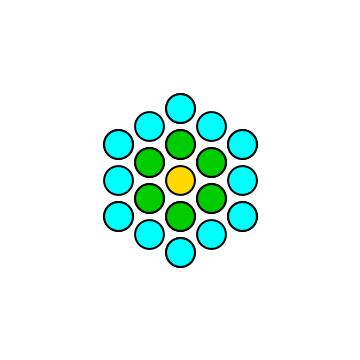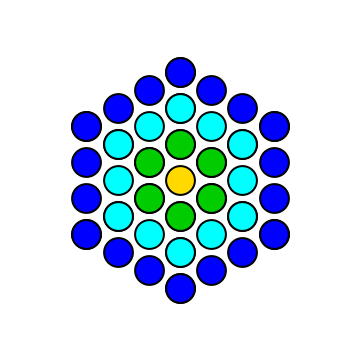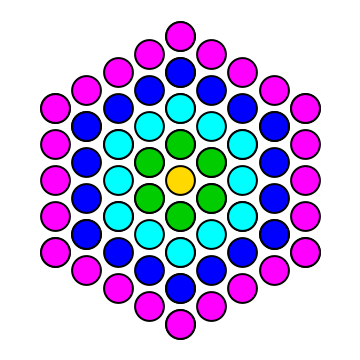1 7 19 37 61 A003215 1 6 12 18 24

#### General pentagonal numbers and partition counts

We also have
(1 − x)(1 − x2)(1 − x3)(1 − x4) ... = 1 − x − x2 + x5 + x7 − x12 − x15 + ...
where the only coefficients are ±1 and the powers of x are the general pentagonal numbers 3k (k ± 1)/2.
and
 1 = 1 + 2 x + 3 x2 + 5 x3 + 7 x4 + 11 x5 + ... + p(n) xn + ... (1 − x)(1 − x2)(1 − x3)(1 − x4) ...

is the generating function for the partition (bags) numbers p(n).
There is a nice, easy proof of this in Algebra, an Elementary Textbook G Chrystal (7th edition, 1964) part II, pages 563-564 using only Ferrer's diagrams which we met earlier.

### Number bases

When we write a number in base 10 or any other base, we are finding a collection of powers of the base which sum to the value. Each power can be absent or repeated a number of times, up to one less than the base.
p( n | parts are powers of base, with repetition, parts chosen from 0..(base-1) )

### Fibonacci Numbers

If we make a line of squares and dominoes (of lengths 1 and 2) allowing repetitions and noting all the different orders of the squares and dominoes, then the number of ways to make a line of length n (sequence with repetition allowed) is as follows:
ncompositions:
sequences with repetition
#
111
21+1, 22
31+1+1, 1+2, 2+13
41+1+1+1, 1+1+2, 1+2+1, 1+3, 2+1+1, 2+25
Can you see why the counts will always be the Fibonacci Numbers? Show why
Consider how we might find all the compositions for 5 using the table above.
EITHER the composition begins with 1
in which case the rest of it is any composition of 4;
OR the composition begins with 2
in which case the rest of it is any composition of 3
These are the only two cases and all compositions of 5 fall into exactly one of these cases.
So the number of compositions of 5 is the number of compositions of 4 (with a 1 in front of each) plus the number of compositions of 3 9with a 2 in front of each).
This applies to any number n: the number of compositions is the sum of the number of compositions of n-1 and of n-2.
Since the number of compositions of 1 is 1 and of 2 is 2 then we have the same starting values as in the Fibonacci series (0,1), 1,2,3,5,8,13,21,...

### Fibonacci again!

Cauchy in 1876 (see Dickson, Volume II, page 130) mentions another way in which the number of compositions are the Fibonacci numbers.
If we do not count any composition which contains the part 1, so that the parts are chosen from 2,3,4,... then the number of compositions on n (sequences, repetition allowed) is a Fibonacci number:
nCompositions#
221
331
44; 2,22
55; 2,3; 3,23
66; 2,4; 3,3; 4,2; 2,2,25
Can you prove why this is so?
Show a hint
If c(n) counts the number of compositions of n using 2,3,4,... with repetition allowed then we need to prove that
c(2)=1, c(3)=1 and c(n)=c(n-1) + c(n-2) for n>3.
Show a proof
If n>3, then any composition (a sequence where the order matters) ends with 2 or ends with a number bigger than 2.
We can take any of the valid compositions of n-1 and increase the final number by 1 so that these will end with a number 3 or more and the new sum will be n.
We can also take any valid compositions of n-2 and append a 2 as an extra part at the end again making the new sum n.
Clearly these actions cannot give the same composition and each gives a composition of n, and all compositions of n must be in one of these two categories (end with a number 3 or bigger or else end with 2).
So c(n) is c(n-1) + c(n-2).

### Palindromic compositions

Another page on this site introduces some nice maths results about numbers that are palindromes as base 10 numbers or in any other base.
But there are some lovely results for compositions (partition sequences) that are palindromic, or in other words, they are the same sequence when reversed. For instance for 7 we have:
1: 1,1,1,1,1,1,1 2: 1,1,3,1,1 3: 1,2,1,2,1 4: 1,5,1 5: 2,1,1,1,2 6: 2,3,2 7: 3,1,3 8: 7
For any composition of n there are always at least 2 palindromic sequences: n 1s and the sequence n itself.
There are some questions to start your investigations into these in the You Do The Maths... section after the Restricted Partitions Calculator below.

### Partitions with maximum product

Which of the partitions (as bags) of n has the maximum product of its parts?
If we take the 7 partitions of 5 and multiplying the parts of each together we have:
• 1 1 1 1 1 with product 1
• 2 1 1 1 has product 2
• 3 1 1 has product 3
• 4 1 and 2 2 1 both have product 4
• 5 has product 5
• 3 2 has the maximum product of 6

#### You Do The Maths ...

1. Find the partitions of 1 to 12 which have the maximum product. Show the answers
nPartitionProduct
111
222
333
44, 224
5326
6339
743, 22312
823318
933327
10223336
11233354
12333381
2. Find a simple method of generating the partition with maximum product for n>4 and also for that product.
(Hint: consider the remainder when n is divided by 3) Show the answers
The partitions with maximum product have parts which are just 2s and 3s.
This is because any part which is 4 or greater can be replaces by 3s and any remainder whose product is larger.

To form the partition: take the maximum number of 3s. There will be a reminder of 0, 1 or 2:
If there is no remainder, the maximum-product partition 3 for all parts .
If there is a remainder of 1, change one of the 3s and the 1 (which has a product of 3) for 2 2 (which has a product of 4). There are two partitions with maximum product, one with 4 as a part and the other 22
If there is a remainder of 2, keep the 2.

The value of the maximum product for n>4 depends on its remainder when divided by 3:
if the remainder is 0: the maximum product is 3n/3;
if the remainder is 1: the maximum product is 22×3(n-4)/3
if the remainder is 2: the maximum product is 2×3(n-2)/3

3. What is the largest product of numbers whose sum is 2020? Show the answer
2020 = 3 ×673 + 1
the largest number is a product of 672 threes and 2 twos (or a 4): 16886...38564 and is 322 digits long!

#### A Maximum-Product Partition Calculator

Here the product notation is useful since it is exactly the product of the parts of a partition.
Maximum-Product Partition C A L C U L A T O R
 a maximum-product partition (bag) partition (bag) with odd parts partition (set) with distinct parts of up to

R E S U L T S: Integer sums Restricted partitions Max Prod partitions Divisors' sum

## Formulas for the Number of Partitions and Compositions

Here are recursive formulas for the 4 types of partition/composition that are used in the Calculators on this page. Both n and k are positive non-zero whole numbers otherwise the result is 0.
The total number of partitions of each kind is the sum over all the part-lengths, that is for k from 1 to n.
We can have a set, multiset (bag) or sequence abbreviated to set, bag, seq. Also we can allow repetition rep or, once used, remove an item from later choices making the choices (parts) distinct (rem).
The recursion equations are used in order with the first to match the given values of n and k determining the result of the function.
The ASSERT expressions are merely for information and are the conditions under which that line will be triggered.
In all cases we have (with f being the function computing the number of collections with sum n and k parts):
• there cannot be more parts k than the sum n. So if n<k then the result is 0:
f(n,k) = 0 if n<k
• There must be at least 1 part so k≥1 otherwise the result is 0:
f(n,k) = 0 if k<1
• a collection (set, bag or sequence) of size k=1 with sum n is always possible and the collection is unique:
f(n,1) = 1
• Alternatively we can replace f(n,1) = 1 with f(0,0) = 1 meaning there is the empty collection which is a partition or composition of 0.
However, it must be the first line in each recursive definition and will have the effect of changing the value of f(0,0) from 0 to 1.
This is the only value of f that is changed for all integer values of n and k (positive, zero and negative).
For example for partition multisets (bags, with repetition allowed):
pbagrep(n, k) = 0 if n<k or k<1
pbagrep(n, 1) = 1 ASSERT n≥k=1
pbagrep(n, k) = pbagrep(n−1, k−1) + pbagrep(n−k, k) ASSERT n≥k>1
becomes
pbagrep(0,0) = 1 ASSERT n = k = 0
pbagrep(n, k) = 0 if n<k or k<1
pbagrep(n, k) = pbagrep(n−1, k−1) + pbagrep(n−k, k) ASSERT n≥k≥1

To find the total number of partitions or compositions we sum the function for k from 1 to n although other methods are indicated in the sections below.

### Accumulating sums

Some recursive formulas are given for the accumulated sums for a given n.
In general, the accumulated sum of f(n,k) is the sum of all values of f(n,i) for i < k:
accf(n, k) = accf(n, k-1) + f(n, k) if k>0; accf(n, 0) = f(n, 0)
The reasoning here is that we take the sum up to the previous value of k, k − 1, and add on the next value of the function for k.
By the same reasoning, to recover the original numbers for individual values of f(n, k) from the accumulated sums we have:
f(n, k) = accf(n, k) − accf(n, k−1) if k>0; f(n, 0) = accf(n, 0)

### Partitions (multisets/bags with repetition)

pbagrep(n, k) is the number of multisets of k positive numbers with sum n:
pbagrep(n, k) = 0 if n<k or k<1
pbagrep(n, 1) = 1 ASSERT n≥k=1
pbagrep(n, k) = pbagrep(n−1, k−1) + pbagrep(n−k, k) ASSERT n≥k>1

A constructive argument for why this recursion works here:
Let the bag be represented as a list of numbers in non-decreasing order (because repetitions are allowed).
A bag (list) of size k and sum n either
begins with 1
so the rest of the bag is any solution for a bag with sum n-1 and length k-1
or begins with a number > 1
so we can find it by increasing every part by 1 in any bag with the sum n-k and k parts
Both of these maintain the invariant that each list is a non-decreasing sequence.

Alternatively, we can also see use the earlier identity that this is the same as counting bags whose sum is n and whose maximum element is k as follows:
The bag contains one part k or else it contains more than one part with value k.

If there is only one part which is k
then the rest of the bag has sum n-k and maximum k-1
there is more than one part which is k
in which case, on removing one of the k parts, we have a bag of n-k and still the maximum part is k

#### Number of partition (bags)

If p(n) denotes the total number of multisets (bags) with sum n, Euler also found that
p(n) = p(n−1) + p(n−2) − p(n−5) − p(n−7) + p(n−12) + p(n−15) − p(n−22) − ...
where p(n) = 0 for n≤0.
The signs alternate by pairs and the numbers subtracted from n are (again!) the General Pentagonal Numbers that we met earlier.

#### An accumulating definition

pbagrepupto(n,k) returns the number of partition bags with sum n and parts less than or equal to k (or, equivalently, of length up to k).
Note that the first line is different. If n>1 then there is always a non-zero result.
The total number of these partitions of n of all sizes is given first at pbagrepupto(n, n) and then for all k > n.
pbagrepupto(n, k) = 0 if n<0 or k< 1
pbagrepupto(n, 1) = 1 ASSERT n≥k=1
pbagrepupto(n, k) = pbagrepuptp(n, k−1) + pbagrepupto(n−k ,k)
A constructive argument for why this recursion works here:
If all the parts are less than k (or the partition is of length less than k)
then these have the same sum n but do not include k:pbagrepupto(n,k-1)
If there is a part k (or the partition is length k)
then it is formed by including a new part k with num n-k and length up to k: pbagrepupto(n-k,k)

### Partitions (sets, distinct numbers)

psetrem(n, k) counts the number of sets of k (distinct) numbers whose sum is n
psetrem(n, k) = 0 if n<k or k<1
psetrem(n, 1) = 1 ASSERT n≥k=1
psetrem(n, k) = psetrem(n−k, k−1) + psetrem(n−k, k) ASSERT n≥k>1

#### Number of partition sets

 The total can be found by summing psetrem(n,k) for k from 1 up to floor( √8n+1 − 1 ) 2
A constructive argument for why this recursion works here:
Let the set be represented as a list of (distinct) numbers in increasing order.
A set (list) with sum n consisting of k members (parts) either contains 1 or does not.
If a set contains 1
by subtracting 1 from each member we will have a set with sum n-k of k-1 non-zero members if we delete the 0 part.
There are psetrem(n-k,k-1) of these.
If it does not contain 1
by reducing each of the k elements by 1 we have a set of k positive members with sum n-k.
There are psetrem(n-k,k) of these.
If all members are positive, by reducing all members by 1 we maintain the set property.

### Compositions (sequences) with repetition

pseqrep(n, k) counts the number of sequences of k positive integers (with repetition allowed) whose sum is n
pseqrep(n, k) = 0 if n<k or k<1
pseqrep(n, 1) = 1 ASSERT n≥k=1
pseqrep(n, k) = pseqrep(n−1, k−1) + pseqrep(n−1, k) ASSERT n≥k>1

This is just the recurrence for the Binomial numbers so that a direct formula is
 pseqrep(n, k) = Binomial(n−1, k−1) = (n−1)! (k−1)! (n−k)!

#### Number of compositions allowing repetition

The total number of compositions of n (with repetition allowed) is 2n-1
A constructive argument for why this recursion works here:
The first item is 1 or is bigger than 1.
If the first part is 1
remove it to get a sequence with sum n-1 of length k-1
If the first part is greater than 1
then it is a sequence with sum n-1 of length k with the first part increased by 1
Since we only alter the first part, items may get repeated.

#### An accumulating version

pseqrepupto(n, k) counts the number of sequences of up to k positive integers (with repetition allowed) whose sum is n. This has the same recursion as the version above except that the boundary conditions are different. Here all the top row (n = 1) is 1 (except for k=0) and sine each row is determined by adding two elements from the row above (row n−1) then this accumulates the entries on each row:
pseqrepupto(n, k) = 0 if n<1 or k< 1
pseqrepupto(1, k) = 1 ASSERT k ≥ 1
pseqrepupto(n, k) = pseqrepupto(n − 1, k − 1) + pseqrepupto(n − 1, k) ASSERT n≥k>1

#### Palindromic compositions

Sequences which are the same when reversed are palindromes.
pseqreppalind(n, k) counts the number of palindromic sequences of length k with sum n.
We cannot have a palindromic composition for an odd number n with even length (see the Things to Do below).

pseqreppalind(n, k) = 0 if n<1 or k< 1 or (n is odd and k is even)
pseqreppalind(n, 1) = 1
pseqreppalind(n, k) = pseqreppalind(n − 1, k − 1) + pseqreppalind(n − 1, k)
A constructive argument for why this recursion works here:
if the length is 1 there is just one palindromic sequence: [n]
if the length is 2 then if n is even there is just one of length 2: [n/2, n/2] but none if n is odd.
if k is even and >2 (and n even) we take any palindrome of n-1 of length k-1 (odd) and increase the central part by 1;
in this case we also see that there are none for n-1 (odd) of length k (even).
If k is odd and >1 we take any palindrome of n-1 of length k-1 (even) and insert a 1 in between the two halves.
Also for all palindromes of n-1 of length k (odd) we n increase the central part by 1.
In both cases (k even and k odd) we have the sum of palindromes of n-1 of length k-1 and of length k.

An algorithm to produce palindromes in increasing lexicographic order from [1,1,1,...,1] to the singleton [n] is:

function pl(n) is defined as:  if n<0 return nothing;  if n=0 return the empty sequence [].  if n>0 for each i from 1 while 2i≤n do:   insert i before the start and after the end of each palindrome returned by calling pl(n-2i)

#### Fibonacci Palindromes

By restricting the choice of parts to {1,2}, the number of composition palindromes of n is again always a Fibonacci number.
n#length
123456789101112
111
2211
31..1
43.111
52..1.1
65..1211
73....2.1
88...12311
95....1.3.1
1013....133411
118......3.4.1
1221.....1364511

### Compositions (sequences) without repetition

pseqrem(n, k) counts the number of sequences of k distinct positive numbers whose sum is n
pseqrem(n, k) = 0 if n<k or k<1
pseqrem(n, 1) = 1 ASSERT n≥k=1
pseqrem(n, k) = pseqrem(n−k, k) + k pseqrem(n−k, k−1) ASSERT n≥k>1
A constructive argument for why this recursion works here:
The sequence contains 1 or all parts are > 1
If k=1
there is only the single sequence n.
if k>1:
if all parts are bigger than 1
then we can get it by adding 1 to every part of any sequence (of distinct parts) of length k with sum n-k and still maintain distinctness of parts.
There are pseqrem(n-k,k) of these.
if the sequence contains 1
we can make it by taking any sequence on n-k parts with sum k-1 and increasing each of the k-1 parts by 1 (to maintain the uniqueness of parts) and then, in each of its k gaps before or after a part, insert a 1.
There are pseqrem(n-k, k-1) such sequences each with k gaps in which to insert the 1.

### Algorithms to generate Partitions and Compositions

We can justify each recursion for each case by a constructive argument, that is one which tells us how to construct the partitions and compositions and so find an algorithm to generate each kind as well as to count them: Show the recursion arguments in the subsections above

## A remarkable connection between partitions (bags) of n and "the sum of the divisors of n"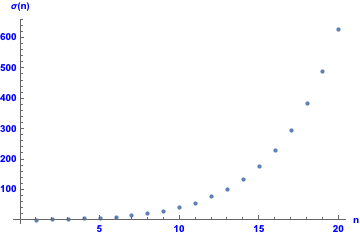Show p plot to n=20: Show p plot to n=50: Show sigma plot to n= 200: Show sigma plot to n=1000:
If p(n) denotes the total number of multisets (bags) with sum n, Euler also found that
p(n) = p(n−1) + p(n−2) − p(n−5) − p(n−7) + p(n−12) + p(n−15) − p(n−22) − ...
where p(n) = 0 for n≤0.
The signs alternate by pairs and the numbers subtracted from n are (again!) the General Pentagonal Numbers that we met earlier.

The recurrence involving the General Pentagonal Numbers has another surprise, again found by Euler.
Let's introduce the sum of all the divisors of a number n which is often denoted using the greek small letter σ.

For example:
σ(10) = the sum of the divisors of 10 = 1 + 2 + 5 + 10 = 18
Since the divisors include 1 and the number itself, σ(n) is always n+1 for the prime numbers and only for the prime numbers.

Yet Euler found that the recurrence relation for partition bags that involves the General Pentagonal Numbers is also exactly the same recurrence as for σ(n), except for the value of σ(0) which is the number itself whereas p(0) is 1 for partition bags:

σ(n) = σ(n−1) + σ(n−2) − σ(n−5) − σ(n−7) + σ(n−12) + σ(n−15) − σ(n−22) − ...
where σ(0) = n if n is a General Pentagonal number itself
and σ(m) = 0 for all negative values m.
For example, computing σ(10) by this formula we have:
σ(10)
= σ(n−1) + σ(n−2) − σ(n−5) − σ(n−7)
= σ(9) + σ(8) − σ(5) − σ(3)
= 13 + 15 − 6 − 4
= 18

Check: Sum of all divisors of 10 is 1 + 2 + 5 + 10 = 18
whereas if we use a General Pentagonal number such as n = 12 we have:
σ(12)
= σ(n−1) + σ(n−2) − σ(n−5) − σ(n−7) + σ(n−12)
= σ(11) + σ(10) − σ(7) − σ(5) + σ(0)
= 12 + 18 − 8 − 6 + 12
= 28

Check: Sum of all divisors of 12 is 1 + 2 + 3 + 4 + 6 + 12 = 28

This is remarkable and unexpected because the function p(n) smoothly increases as n increases whereas σ(n) varies up and down from one number to the next as shown in the graph on the right. The maximal values of n are those for which σ(n) exceeds σ(k) for all k<n. They are
n = 1, 2, 3, 4, 6, 8, 10, 12, 16, 18, 20, 24, 30, ... A002093.
The minimal numbers for sigma are the primes since σ(n) is always at least 1 + n for divisors 1 and n and equals this minumum only for the prime numbers n.

### Sum of Divisors Calculator

This Calculator will help you explore the Sum Of Divisors function σ(n) and the recurrence relation that it shares with the partitions counting function p(n).
Sum of Divisors C A L C U L A T O R
 σ( up to )
R E S U L T S: Integer sums Restricted partitions Max Prod partitions Divisors' sum

### Two more recurence relations connecting p(n) and σ(n)

Here is another connection between σ and p again using the General Pentagonal numbers:
σ(n) = p(n−1) + 2 p(n−2) − 5 p(n−5) − 7 p(n−7) + 12 p(n−12) + 15 p(n−15) − 22 p(n−22) − ...
where p(n) = 0 for n≤0
The following identity connects p and σ directly without using the general pentagonals:
n p(n) = σ(1) p(n−1) + σ(2) p(n−2) + σ(3) p(n−3) + ... +σ(n) p(0)
To quote from the Surprising Connections paper listed in the References below
Our discussion is over, but we sense that the mystery is only partially revealed.
How can the problem of expressing a number as a sum, be at all related to expressing that same number as a product?
Euler was astonished at these results

• Integer Partitions G E Andrews, K Eriksson (Cambridge 2010 edition) is an excellent little paperback with many examples of Partition Identities and proofs.
• Theory of Partitions G E Andrews, (Cambridge Uni press, 1998 paperback edition)
This is now the classic text on Partitions and well worth getting if you are interested in Partitions, though much of the maths is at degree level. It was first published in 1984 but the 1998 paperback version includes recent results up to that date.
• How Euler Did It by C E Sandifer, (Math Assoc of America, 2007)
Chapter 14 is on Philipe Naudé's problem and on the GF for the number of partitions of n of length k.
The book is well worth reading to see how Euler's developed so many important results in so many areas of mathematics.
• Memoir on the Theory of the Compositions of a Number P A MacMahon (1893) Phil Trans Royal Soc A pages 835-901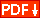The first book on compositions of an integer (sequences) as opposed to partitions (where the order of the parts is immaterial). He also introduces palindromic compositions. After this work not much was published on compositions until the 1950s.
• A Note on Partitions and Triangles With Integer Sides G E Andrews, Amer Math Monthly vol 86 (1979) pages 477-478.
This proves a slightly different formulation for T(n) that we met in the Alcuin's sequence problem above, namely that
 floor(n/2) T(n) = p3(n) − Σ p2(j) j = 1
where pk(n) is the number of partitions of n into k parts
floor(x) is the nearest integer less than x if x is not a whole number otherwise it is x itself.
and another remarkably simple equation which does not use the number of partitions:
T(n) = round( n2/12 ) − floor(n/4) floor((n+2)/4)
where round(x) is the integer nearest to x.
• History of the Theory of Numbers: Vol II Diophantine Analysis by L E Dickson
is a classic and monumental reference work on all aspects of the History and development of Number Theory, in 3 volumes (Vol 1 Divisibility and Primality and volume III on Quadratic and Higher Forms). The original edition was 1920 but Dover reprinted it in 2005 with no changes. Nothing since 1920 is covered in the book but it is still a comprehensive summary of useful, fundamental results and a historical survey of references to the famous and not-so-famous mathematicians who have developed the area of mathematics we now call Number Theory. The link above is to a new cheap Dover paperback edition (2005) of Volume II where chapter 3 is on Partitions from Euler to MacMahon.
• Surprising Connections between Partitions and Divisors T J Osler, A Hassen, T R Chandrupatla The College Mathematics Journal 38 (Sep 2007), pages 278-287 JSTOR pdf
Explains and proves the recursion equation that Euler discovered for both the partition (bags) and the sum of the divisors of a number are the same apart from the base cases.
• Some Sequences of Integers P J Cameron Discrete Mathematics 75 (1989) pages 89-102 abstract and pdf
This academic paper explains how partitions relate to compositions via GFs as an example of a far more general approach to ordered versus unordered collections and their counting sequences.
• A link to a video lecture by Prof Ken Ono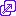(2011) on the background to his new exact formula for the partition numbers aimed at a general audience (70 minutes). Highly recommended.© 2019 Dr Ron Knott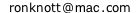Dr Knott's Maths Fibonacci and HOME page
created: 10 October 2019,